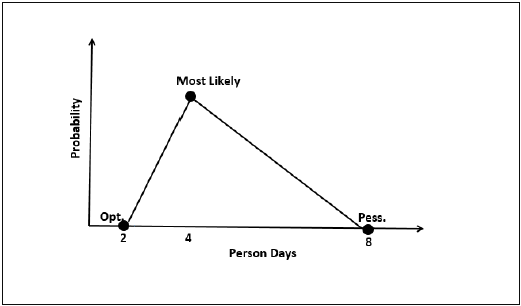# Estimation Techniques - Three Point

Three-point Estimation looks at three values −

• the most optimistic estimate (O),
• a most likely estimate (M), and
• a pessimistic estimate (least likely estimate (L)).

There has been some confusion regarding Three-point Estimation and PERT in the industry. However, the techniques are different. You will see the differences as you learn the two techniques. Also, at the end of the PERT technique, the differences are collated and presented. If you want to look at them first, you can.

Three-point Estimate (E) is based on the simple average and follows triangular distribution.

E = (O + M + L) / 3## Standard Deviation

In Triangular Distribution,

Mean = (O + M + L) / 3

Standard Deviation = √ [((O − E)2 + (M − E)2 + (L − E)2) / 2]

### Three-point Estimation Steps

Step 1 − Arrive at the WBS.

Step 2 − For each task, find three values − most optimistic estimate (O), a most likely estimate (M), and a pessimistic estimate (L).

Step 3 − Calculate the Mean of the three values.

Mean = (O + M + L) / 3

Step 4 − Calculate the Three-point Estimate of the task. Three-point Estimate is the Mean. Hence,

E = Mean = (O + M + L) / 3

Step 5 − Calculate the Standard Deviation of the task.

Standard Deviation (SD) = √ [((O − E)2 + (M − E)2 + (L - E)2)/2]

Step 6 − Repeat Steps 2, 3, 4 for all the Tasks in the WBS.

Step 7 − Calculate the Three-point Estimate of the project.

E (Project) = ∑ E (Task)

Step 8 − Calculate the Standard Deviation of the project.

SD (Project) = √ (∑SD (Task)2)

## Convert the Project Estimates to Confidence Levels

The Three-point Estimate (E) and the Standard Deviation (SD) thus calculated are used to convert the project estimates to “Confidence Levels”.

The conversion is based such that −

• Confidence Level in E +/– SD is approximately 68%.
• Confidence Level in E value +/– 1.645 × SD is approximately 90%.
• Confidence Level in E value +/– 2 × SD is approximately 95%.
• Confidence Level in E value +/– 3 × SD is approximately 99.7%.

Commonly, the 95% Confidence Level, i.e., E Value + 2 × SD, is used for all project and task estimates.

## Useful Video Courses

Video

#### Quantity Surveying/Building Estimation With Cad And Excel

136 Lectures 12.5 hours